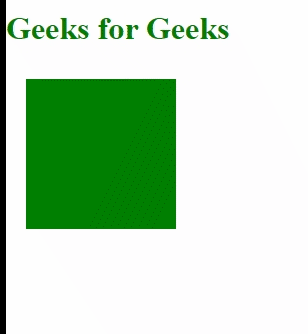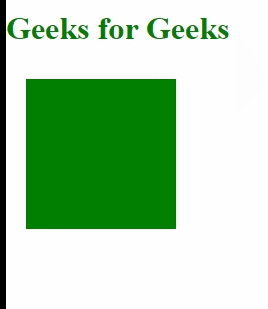Related Articles

# D3.js easePolyOut Function

• Last Updated : 07 Sep, 2020

The d3.easePolyOut() function in d3.js is used for reverse exponential easing transition effect to a particular element. If the exponent is not specified then it defaults to three.

Approach: The D3.js transition function will be used for applying different easing effects on a particular element. First of all, create an SVG element and append it to the body of the HTML page, then create a rectangle or any other shape and append it to the SVG. Set some attributes of the applied shape to give it nice color and size and apply d3.transition function followed by ease() function and give the d3.easePolyOut as a parameter to ease function.

Hey geek! The constant emerging technologies in the world of web development always keeps the excitement for this subject through the roof. But before you tackle the big projects, we suggest you start by learning the basics. Kickstart your web development journey by learning JS concepts with our JavaScript Course. Now at it's lowest price ever!

Syntax:

```d3.easePolyOut
```

or

``` d3.easePolyOut.exponent(t);
```

Parameter: This function accepts a single parameter as mentioned above and described below.

• t: It is the time that is to be raised to the power of the exponent.

Return Values: This function does not return anything.

Below given are a few examples of the function given above.

Example 1:

## HTML

 ```<``html` `lang``=``"en"``>`` ` `<``head``>``    ``<``meta` `charset``=``"UTF-8"``>``    ``<``meta` `name``=``"viewport"` `content="``        ``width``=``device``-width, ``initial-scale``=``1``.0">`` ` `    ``<``script` `type``=``"text/javascript"` `        ``src``=``"https://d3js.org/d3.v4.min.js"``>``    `````` ` `<``body``>``    ``<``h1` `style``=``"color:green"``>Geeks for Geeks``    ``<``svg` `width``=``"500px"` `height``=``"500px"``>``     ` `    ``<``script``>``        ``var svg = d3.select("svg")``            ``.attr("transform", "translate(0, -50)");``        ``svg.append("rect")``            ``.attr("x", 20)``            ``.attr("y", 60)``            ``.attr("width", 150)``            ``.attr("height", 150)``            ``.attr("fill", "green")``            ``.transition()``            ``.ease(d3.easePolyOut)``            ``// .delay(6000)``            ``.attr("x", 100)``            ``.attr("y", 100)``            ``.attr("width", 10)``            ``.attr("height", 10)``            ``.duration(2000);``    `````` ` ``

Output:Example 2:

## HTML

 ```<``html` `lang``=``"en"``>`` ` `<``head``>``    ``<``meta` `charset``=``"UTF-8"``>``    ``<``meta` `name``=``"viewport"` `content="``  ``width``=``device``-width, ``initial-scale``=``1``.0">````<``script` `type``=``"text/javascript"` `src``=``    ``"https://d3js.org/d3.v4.min.js"``>```` ` `<``body``>``    ``<``h1` `style``=``"color:green"``> Geeks for Geeks``    ``<``svg` `width``=``"500px"` `height``=``"500px"``>``     ` `    ``<``script``>``        ``var svg = d3.select("svg")``            ``.attr("transform", "translate(0, -50)");``        ``svg.append("rect")``            ``.attr("x", 20)``            ``.attr("y", 60)``            ``.attr("width", 150)``            ``.attr("height", 150)``            ``.attr("fill", "green")``            ``.transition()``            ``.ease(d3.easePolyOut.exponent(.1))``            ``// .delay(6000)``            ``.attr("x", 100)``            ``.attr("y", 100)``            ``.attr("width", 10)``            ``.attr("height", 10)``            ``.duration(2000);``    `````` ` ``

Output:My Personal Notes arrow_drop_up